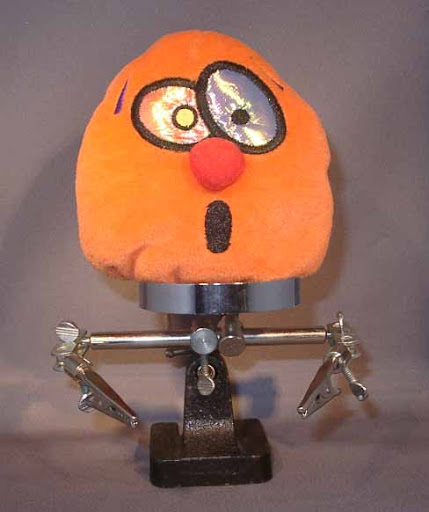## Saturday, 22 September 2007

### Temperature drop

My extruder controller is working much better after I cured the motor noise problem. The 10 bit SAR ADC also seems to work better than the 16 bit sigma-delta version did. With the 16 bit one there was a lot of noise on the readings, even when the motor wasn't running. I had to average over many samples to get a consistent reading which delayed the response. With the 10 bit ADC I just read it and compare it with the temperature set point value to decide if the heater should be on or off. That gives a temperature swing of about ± 3°C with the heater going on and off every four or five seconds.

The temperature is calculated from the ADC reading and vice versa by the PC with the following Python class :-
from math import *class Thermistor: "Class to do the thermistor maths" def __init__(self, r0, t0, beta, r1, r2):     self.r0 = r0                        # stated resistance     self.t0 = t0 + 273.15               # temperature at stated resistance, e.g. 25C     self.beta = beta                    # stated beta     self.vref = 1.5 * 1.357 / 1.345     # ADC reference, corrected     self.vcc = 3.3                      # supply voltage to potential divider     self.vs = r1 * self.vcc / (r1 + r2) # effective bias voltage     self.rs = r1 * r2 / (r1 + r2)       # effective bias impedance     self.k = r0 * exp(-beta / self.t0)  # constant part of calculation def temp(self,adc):     "Convert ADC reading into a temperature in Celsius"     v = adc * self.vref / 1024          # convert the ADC value to a voltage     r = self.rs * v / (self.vs - v)     # resistance of thermistor     return (self.beta / log(r / self.k)) - 273.15        # temperature def setting(self, t):     "Convert a temperature into a ADC value"     r = self.r0 * exp(self.beta * (1 / (t + 273.15) - 1 / self.t0)) # resistance of the thermistor     v = self.vs * r / (self.rs + r)       # the voltage at the potential divider     return round(v / self.vref * 1024)    # the ADC reading
It is instantiated as follows :-
thermistor = Thermistor(10380, 21, 3450, 1790, 2187)
10380 is the resistance of the thermistor measured by my multimeter at a room temperature of 21°C. 3450 is the beta of the thermistor taken from the data sheet. The last two values are the two resistors forming a potential divider with the thermistor wired across the second one, again the values are measured with a multimeter. The fudge factor of 1.357 / 1.345 corrects the MSP430 internal reference voltage so that it agrees with the multimeter.

The result seems to track the temperature measured by a thermocouple to within about 5°C, good enough for me. Just as I had finished checking it, I knocked the thermistor leads with my thermocouple and it fell off. It was stuck to the brass nozzle with JB Weld but I forgot to roughen the surface first. I am now waiting 16 hours for it to set again.

The extruder controller firmware is only about 400 lines of C. As well as temperature control it also controls the DC motor precisely using the shaft encoder and handles the I²C protocol.

I have now completed all the mechanical parts, the electronics and the firmware. I just need to get the RepRap host code to talk to my non standard hardware to complete the machine.

1.Hey,

I love reading your posts... you're very knowledgeable. Keep up the good work.

Its cool that you're going to try and use the RepRap software to drive your machine, i think thats a really good idea.

It would be cool if we could modify it in such a way that there is an interface class to each toolhead. This should be done in such a way that to update the code to allow it to drive an extruder (or cartesian bot) that uses different electronics, you simply define the specific class.

for example: we could have a CartesianInterface class that can be extended and implemented in such a way that it sends the proper commands to the boards. we would then have a DarwinCartesianBot class, NopHeadCartesianBot, etc. then in the preferences, you just specify what electronics you are using and it would know the right class to instantiate and use.

i'm pretty sure that the software is already setup to support something like this. you'll definitely have to get in there and dig around to find out the extent.

good luck!

2.Hi Zach,
Thanks for the encouragement, I think I am going to need it. I am not looking forward to grappling with Eclipse and Java.